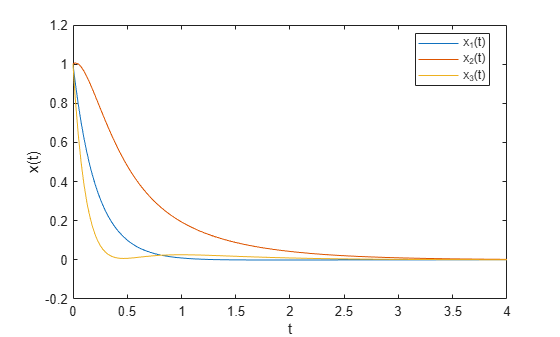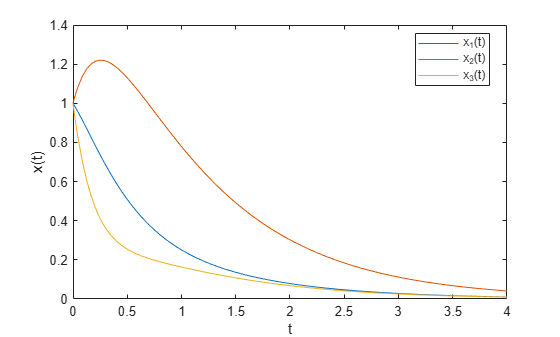## Multi-Objective Goal Attainment Optimization

This example shows how to solve a pole-placement problem using the multiobjective goal attainment method. This algorithm is implemented in the function `fgoalattain`.

### Equation that Describes Evolution of System

Consider a 2-input 2-output unstable plant. The equation describing the evolution of the system x(t) is

`$\frac{dx}{dt}=Ax\left(t\right)+Bu\left(t\right),$`

where u(t) is the input (control) signal. The output of the system is

`$y\left(t\right)=Cx\left(t\right).$`

The matrices A, B, and C are

```A = [ -0.5 0 0; 0 -2 10; 0 1 -2 ]; B = [ 1 0; -2 2; 0 1 ]; C = [ 1 0 0; 0 0 1 ];```

### Optimization Objective

Suppose that the control signal u(t) is set as proportional to the output y(t):

`$u\left(t\right)=Ky\left(t\right)$`

for some matrix K.

This means that the evolution of the system x(t) is:

`$\frac{dx}{dt}=Ax\left(t\right)+BKCx\left(t\right)=\left(A+BKC\right)x\left(t\right).$`

The object of the optimization is to design K to have the following two properties:

1. The real parts of the eigenvalues of (A + B*K*C) are smaller than [-5, -3, -1]. (This is called pole placement in the control literature.)

2. abs(K) <= 4 (each element of K is between -4 and 4)

In order to solve the optimization, first set the multiobjective goals:

`goal = [-5, -3, -1];`

Set the weights equal to the goals to ensure same percentage under- or over-attainment in the goals.

`weight = abs(goal);`

Initialize the output feedback controller

`K0 = [ -1 -1; -1 -1];`

Set upper and lower bounds on the controller

`lb = repmat(-4,size(K0)) `
```lb = 2×2 -4 -4 -4 -4 ```
`ub = repmat(4,size(K0))`
```ub = 2×2 4 4 4 4 ```

Set optimization display parameter to give output at each iteration:

`options = optimoptions('fgoalattain','Display','iter');`

Create a vector-valued function eigfun that returns the eigenvalues of the closed loop system. This function requires additional parameters (namely, the matrices A, B, and C); the most convenient way to pass these is through an anonymous function:

`eigfun = @(K) sort(eig(A+B*K*C));`

### Call Optimization Solver

To begin the optimization we call FGOALATTAIN:

```[K,~,attainfactor] = ... fgoalattain(eigfun,K0,goal,weight,[],[],[],[],lb,ub,[],options);```
``` Attainment Max Line search Directional Iter F-count factor constraint steplength derivative Procedure 0 6 0 1.88521 1 13 1.031 0.02998 1 0.745 2 20 0.3525 0.06863 1 -0.613 3 27 -0.1706 0.1071 1 -0.223 Hessian modified 4 34 -0.2236 0.06654 1 -0.234 Hessian modified twice 5 41 -0.3568 0.007894 1 -0.0812 6 48 -0.3645 0.000145 1 -0.164 Hessian modified 7 55 -0.3645 0 1 -0.00515 Hessian modified 8 62 -0.3675 0.0001549 1 -0.00812 Hessian modified twice 9 69 -0.3889 0.008327 1 -0.00751 Hessian modified 10 76 -0.3862 0 1 0.00568 11 83 -0.3863 1.801e-13 1 -0.998 Hessian modified twice Local minimum possible. Constraints satisfied. fgoalattain stopped because the size of the current search direction is less than twice the value of the step size tolerance and constraints are satisfied to within the value of the constraint tolerance. ```

The value of the control parameters at the solution is:

`K`
```K = 2×2 -4.0000 -0.2564 -4.0000 -4.0000 ```

The eigenvalues of the closed loop system are in eigfun(K) as follows: (they are also held in output fval)

`eigfun(K)`
```ans = 3×1 -6.9313 -4.1588 -1.4099 ```

The attainment factor indicates the level of goal achievement. A negative attainment factor indicates over-achievement, positive indicates under-achievement. The value attainfactor we obtained in this run indicates that the objectives have been over-achieved by almost 40 percent:

`attainfactor`
```attainfactor = -0.3863 ```

### Evolution of System Via Solution to ODE

Here is how the system x(t) evolves from time 0 to time 4, using the calculated feedback matrix K, starting from the point x(0) = [1;1;1].

First solve the differential equation:

`[Times, xvals] = ode45(@(u,x)((A + B*K*C)*x),[0,4],[1;1;1]);`

Then plot the result:

```plot(Times,xvals) legend('x_1(t)','x_2(t)','x_3(t)','Location','best') xlabel('t'); ylabel('x(t)');```### Set Goals To Be Achieved Exactly

Suppose we now require the eigenvalues to be as near as possible to the goal values, [-5, -3, -1]. Set options.GoalsExactAchieve to the number of objectives that should be as near as possible to the goals (i.e., do not try to over-achieve):

All three objectives should be as near as possible to the goals.

`options.GoalsExactAchieve = 3;`

### Call Optimization Solver

We are ready to call the optimization solver:

```[K,fval,attainfactor,exitflag,output,lambda] = ... fgoalattain(eigfun,K0,goal,weight,[],[],[],[],lb,ub,[],options);```
``` Attainment Max Line search Directional Iter F-count factor constraint steplength derivative Procedure 0 6 0 1.88521 1 13 1.031 0.02998 1 0.745 2 20 0.3525 0.06863 1 -0.613 3 27 0.1528 -0.009105 1 -0.22 Hessian modified 4 34 0.02684 0.03723 1 -0.166 Hessian modified 5 41 1.388e-17 0.005703 1 -0.116 Hessian modified 6 48 4.963e-19 9.592e-06 1 -1.15e-15 Hessian modified 7 55 3.388e-21 4.601e-11 1 -2.61e-14 Hessian modified Local minimum possible. Constraints satisfied. fgoalattain stopped because the size of the current search direction is less than twice the value of the step size tolerance and constraints are satisfied to within the value of the constraint tolerance. ```

The value of the control parameters at this solution is:

`K`
```K = 2×2 -1.5953 1.2040 -0.4201 -2.9047 ```

This time the eigenvalues of the closed loop system, which are also held in output fval, are as follows:

`eigfun(K)`
```ans = 3×1 -5.0000 -3.0000 -1.0000 ```

The attainment factor is the level of goal achievement. A negative attainment factor indicates over-achievement, positive indicates under-achievement. The low attainfactor obtained indicates that the eigenvalues have almost exactly met the goals:

`attainfactor`
```attainfactor = 3.3879e-21 ```

### Evolution of New System Via Solution to ODE

Here is how the system x(t) evolves from time 0 to time 4, using the new calculated feedback matrix K, starting from the point x(0) = [1;1;1].

First solve the differential equation:

`[Times, xvals] = ode45(@(u,x)((A + B*K*C)*x),[0,4],[1;1;1]);`

Then plot the result:

```plot(Times,xvals) legend('x_1(t)','x_2(t)','x_3(t)','Location','best') xlabel('t'); ylabel('x(t)');```Watch now